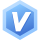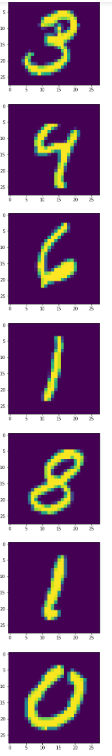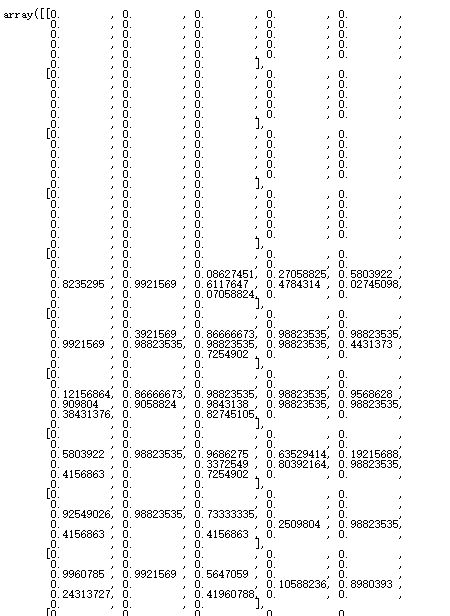# 用CNN卷积神经网络识别手写数字（MNIS 厂数据集）

• 写回答

#### 2条回答默认 最新

•关注
• 这篇博客: mnist手写数字体识别CNN训练测试完美复现，以及自己手写数字进行测试中的 1、mnist数据集介绍 部分也许能够解决你的问题, 你可以仔细阅读以下内容或跳转源博客中阅读:
• 这个数据集是来自美国国家标准与技术研究所, National Institute of Standards and Technology (NIST)。训练集 (training set) 由来自 250 个不同人手写的数字构成, 其中 50% 是高中学生, 50% 来自人口普查局 (the Census Bureau) 的工作人员. 测试集(test set) 也是同样比例的手写数字数据。

为什么要找这么多人来写呢？就是要增强学习结果的泛化能力，避免最后只认识某几个人的手写体。

``````import pylab
print('训练数据：',data.train.images)
print('训练集尺寸：',data.train.images.shape)
print('训练集标签尺寸：',data.train.labels.shape)
print('测试集尺寸：',data.test.images.shape)
print('测试集标签尺寸：',data.test.labels.shape)
'''
回归：
训练数据： [[0. 0. 0. ... 0. 0. 0.]
[0. 0. 0. ... 0. 0. 0.]
[0. 0. 0. ... 0. 0. 0.]
...
[0. 0. 0. ... 0. 0. 0.]
[0. 0. 0. ... 0. 0. 0.]
[0. 0. 0. ... 0. 0. 0.]]
训练集尺寸： (55000, 784)
训练集标签尺寸： (55000, 10)
测试集尺寸： (10000, 784)
测试集标签尺寸： (10000, 10)
'''
``````

可以看到，在这个数据集中，训练集一共有55000个图片，存放在一个矩阵数组中，每一行是一个图片。测试机一共有10000个图片。他们对应的标签就是one_hot编码，例如数字0的标签是1000000000，数字1的标签是0100000000，以此类推。

打印几张mnist中的图片来看一下：

``````import pylab
import tensorflow as tf
from tensorflow.examples.tutorials.mnist import input_data
DATA_DIR = 'MNIST_data'
pylab.figure(figsize=(10,10))
for i in range(10):
im = data.train.images[i]
im = im.reshape((28,28))
pylab.imshow(im)
pylab.show()
im
``````这个数字。。。有的连我自己都不认识。

每一个图片是这样保存的，都是一个28*28的数组。• 以下回答由chatgpt基于相关博客总结生成:

首先，感谢您对CNN卷积神经网络的学习和使用，下面是我对该问题的解答和建议：

1. 数据集 首先需要下载MNIST数据集，可以使用TensorFlow提供的API进行下载，也可以到官网进行下载。下载完毕后可以采用TensorFlow提供的读取程序对数据集进行读取操作。

2. 模型设计 CNN模型的设计主要包括卷积层、池化层、全连接层和输出层。这里建议使用TensorFlow框架，可以参考TensorFlow官网提供的样例进行操作。

3. 模型训练 对于模型训练，可以采用TensorFlow提供的优化器进行梯度下降的计算。同时也需要对训练集和测试集进行分离，可以采用随机打乱的方式进行。

4. 模型评估 在模型评估方面，可以采用测试集对训练好的模型进行评估和检验，主要包括准确率、精确率和召回率等指标，可以使用confusion_matrix函数进行计算。

5. 代码实现 对于代码实现方面，在TensorFlow框架下可以采用以下代码进行实现：

``````# 导入相关包
import tensorflow as tf
from tensorflow.examples.tutorials.mnist import input_data

# 读取MNIST数据集

# 设计卷积神经网络模型
x = tf.placeholder(tf.float32, [None, 784])
y_true = tf.placeholder(tf.float32, [None, 10])

x_reshape = tf.reshape(x, [-1, 28, 28, 1])
conv1 = tf.layers.conv2d(inputs=x_reshape, filters=32, kernel_size=[5, 5], padding="same", activation=tf.nn.relu)
pool1 = tf.layers.max_pooling2d(inputs=conv1, pool_size=[2, 2], strides=2)
conv2 = tf.layers.conv2d(inputs=pool1, filters=64, kernel_size=[5, 5], padding="same", activation=tf.nn.relu)
pool2 = tf.layers.max_pooling2d(inputs=conv2, pool_size=[2, 2], strides=2)
pool2_flat = tf.reshape(pool2, [-1, 7 * 7 * 64])
dense = tf.layers.dense(inputs=pool2_flat, units=1024, activation=tf.nn.relu)
dropout = tf.layers.dropout(inputs=dense, rate=0.4)
y_pred = tf.layers.dense(inputs=dropout, units=10)

# 定义损失函数
cross_entropy = tf.reduce_mean(tf.nn.softmax_cross_entropy_with_logits(logits = y_pred, labels = y_true))

# 定义优化器进行梯度下降计算

# 评估模型准确率
correct_prediction = tf.equal(tf.argmax(y_pred, 1), tf.argmax(y_true, 1))
accuracy = tf.reduce_mean(tf.cast(correct_prediction, tf.float32))

# 训练模型并测试模型准确率
with tf.Session() as sess:
sess.run(tf.global_variables_initializer())
for i in range(2000):
batch_xs, batch_ys = mnist.train.next_batch(100)
sess.run(train_step, feed_dict = {x: batch_xs, y_true: batch_ys})
if i % 100 == 0:
acc = sess.run(accuracy, feed_dict = {x: batch_xs, y_true: batch_ys, keep_prob: 1.0})
print("step %d, training accuracy %g" % (i, acc))

# 对模型进行测试
test_acc = sess.run(accuracy, feed_dict = {x: mnist.test.images, y_true: mnist.test.labels})
print("test accuracy %g" % test_acc)
``````

希望对您有所帮助，如有任何疑问可随时在评论区提出，感谢您的阅读。

评论

#### 悬赏问题

• ¥15 fpga二选一数据选择器语句分析
• ¥15 matlab有svec这个函数吗？
• ¥15 无法调用VideoWriter_fourcc
• ¥15 VB6.0无法加载网页验证码图片到picturebox中，求解。
• ¥15 C#和GDAL对栅格处理
• ¥15 我现在有一些关于提升机故障的专有文本数据，量也不多，我在label studio上进行了关系和实体的标注，完成了知识图谱的构造，那么我使用生成式模型的话，我能做哪些工作来写我的论文？
• ¥15 电脑连不上无线网络如下诊断反馈应该如何操作
• ¥15 telegram api 使用forward_messages方法转发消息时，目标群组里面会出现此消息来源，如何隐藏？
• ¥15 关于#tensorflow#的问题：有没有什么方法可以让机器自己学会像素风格的图片
• ¥15 Oracle触发器字段变化时插入指定值